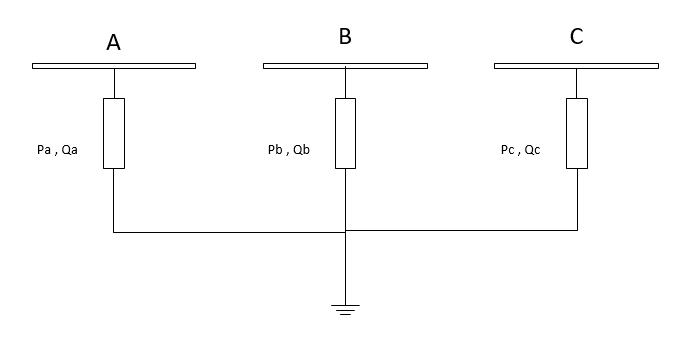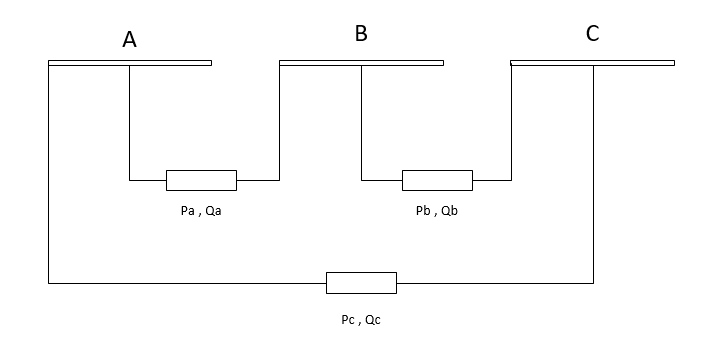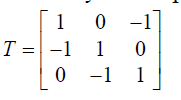Note

Loads should always have a positive p_mw value, since all power values are given in the consumer system. If you want to model constant generation, use a Static Generator (sgen element) instead of a negative load. With Asymmetric Load three phase load values can be supplied seperately

## Create Function¶

pandapower.create_asymmetric_load(net, bus, p_a_mw=0, p_b_mw=0, p_c_mw=0, q_a_mvar=0, q_b_mvar=0, q_c_mvar=0, sn_mva=nan, name=None, scaling=1.0, index=None, in_service=True, type='wye')

All loads are modelled in the consumer system, meaning load is positive and generation is negative active power. Please pay attention to the correct signing of the reactive power as well.

INPUT:

net - The net within this load should be created

bus (int) - The bus id to which the load is connected

OPTIONAL:

p_a_mw (float, default 0) - The real power for Phase A load

p_b_mw (float, default 0) - The real power for Phase B load

p_c_mw (float, default 0) - The real power for Phase C load

• negative value -> generation

q_a_mvar float, default 0) - The reactive power for Phase A load

q_b_mvar float, default 0) - The reactive power for Phase B load

q_c_mvar (float, default 0) - The reactive power for Phase C load

sn_kva (float, default: None) - Nominal power of the load

name (string, default: None) - The name for this load

scaling (float, default: 1.) - An OPTIONAL scaling factor to be set customly

type (string,default: wye) - type variable to classify three ph load: delta/wye

index (int,default: None) - Force a specified ID if it is available. If None, the index one higher than the highest already existing index is selected.

in_service (boolean) - True for in_service or False for out of service

OUTPUT:
index (int) - The unique ID of the created element
EXAMPLE:
create_asymmetric_load(net, bus=0, p_c_mw = 9., q_c_mvar = 1.8)

Creates a single phase wye type load

## Input Parameters¶

 Parameter Datatype Value Range Explanation name string name of the load bus * integer index of connected bus p_a_mw* float $$\geq 0$$ Phase A active power of the load [MW] p_b_mw* float $$\geq 0$$ Phase B active power of the load [MW] p_c_mw* float $$\geq 0$$ Phase C active power of the load [MW] q_a_mvar* float Phase A reactive power of the load [MVar] q_b_mvar* float Phase B reactive power of the load [MVar] q_c_mvar* float Phase C reactive power of the load [MVar] const_z_percent* float $$[0,100]$$ percentage of p_mw and q_mvar that is associated to constant impedance load at rated voltage [$$\%$$] const_i_percent* float $$[0,100]$$ percentage of p_mw and q_mvar that is associated to constant current load at rated voltage [$$\%$$] sn_mva float $$>0$$ rated power of the load [kVA] scaling * float $$\geq 0$$ scaling factor for active and reactive power in_service* boolean True / False specifies if the load is in service. type* String wye/delta Connection Type of 3 Phase Load(Valid for three phase load flow only) controllable** bool States if load is controllable or not, load will not be used as a flexibilty if it is not controllable max_p_mw** float Maximum active power min_p_mw** float Minimum active power max_q_mvar** float Maximum reactive power min_q_mvar** float Minimum reactive power

*necessary for executing a power flow calculation.

Note

• The apparent power value sn_mva is provided as additional information for usage in controller or other applications based on panadapower. It is not considered in the power flow!
• ‘wye’ is the same as PH-E loads
• For ‘delta’ loads line-earth voltages and powers are converted to line-line values

## Electric Model¶

Loads are modelled as PQ-buses in the power flow calculation.Even though power values are entered as Line-ground $$P_{a},Q_{a}$$, for delta loads, Power values are actually line-line powers i.e. $$P_{ab},Q_{ab}$$

So, in the algorithm :

Line-ground voltages $$V_{a}$$ are converted to line-line voltages $$V_{ab}$$. Line-Line currents are then converted to Line-ground currents $$I_{a}$$.

$$I_{a}= T. \frac{S_{ab}}{(V_{an}-V_{bn})}$$

$$I_{b}= T. \frac{S_{bc}}{(V_{bn}-V_{cn})}$$

$$I_{c}= T. \frac{S_{ca}}{(V_{cn}-V_{an})}$$

Where